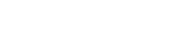# math.acos

`FLOATmath.acosFLOATx`

Available inall subroutines.

Computes the principal value of the arc cosine of its argument `x`.

## Parameters

`x` - Floating point value. The value of `x` should be in the range -1 to 1 inclusive.

## Return Value

Upon successful completion, this function returns the arc cosine of `x` in the range 0 to `math.PI` radians inclusive.

If `x` is `math.NAN`, a NaN will be returned.

If `x` is +1, +0 will be returned.

If `x` is `math.POS_INFINITY` or `math.NEG_INFINITY`, a domain error occurs and a NaN will be returned.

For finite values of x not in the range -1 to 1 inclusive, a domain error occurs and a NaN will be returned.

## Errors

If the `x` argument is finite and is not in the range -1 to 1 inclusive, or is `math.POS_INFINITY` or `math.NEG_INFINITY`, then `fastly.error` will be set to `EDOM`.

## Example

``````declare local var.fo FLOAT;
set var.fo = math.cos(1.1); // Returns math.NAN
if (fastly.error) {  set resp.http.acos-error = fastly.error; // Returns "EDOM"}``````

BETA